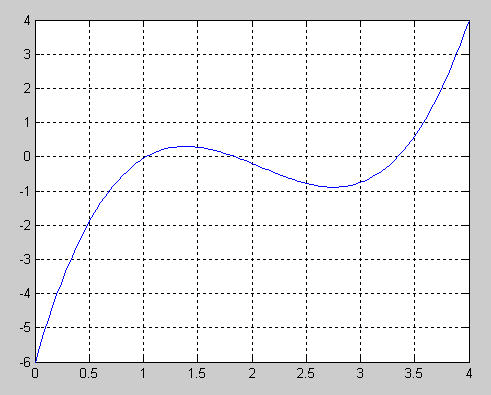Problem

# Determine the highest real root of f (x) = 0.95x3 – 5.9x2 + 10.9x – 6:(a) Graphically.(b)...

Determine the highest real root of f (x) = 0.95x3 – 5.9x2 + 10.9x – 6:

(a) Graphically.

(b) Using the Newton-Raphson method (three iterations, xi = 3.5).

(c) Using the secant method (three iterations, xi–1 = 2.5 and xi = 3.5).

(d) Using the modified secant method (three iterations, xi = 3.5, δ = 0.01).

(e) Determine all the roots with MATLAB.

#### Step-by-Step Solution

Solution 1

a. Type the following code on the console.

Code:

>> x = linspace(0,4);

>> y = 0.95*x.^3-5.9*x.^2+10.9*x-6;

>> plot(x,y);grid

Output:Highest real root ≈ 3.3

b. Newton-Raphson Method

Perform the iterations to get the following table

 i x i f ( x ) f ' ( x ) εa 0 1 2 3 3.5 3.365 6 51 3.345 1 12 3.344 6 45 0.60625 4.5125 0.071249 3.468997 3.992% 0.001549 3.318537 0.614% 7.92E-07 3.315145 0.014%

c. Secant Method

Perform the iterations to get the following table

 i x i −1 f(xi−1) x i f ( x i ) εa 0 2.5 -0.78125 1 3.5 0.60625 2 3.063063 -0.6667 3 3.291906 -0.16487 3.5 3.063 0 63 3.291 9 06 3.367 0 92 0.60625 -0.6667 14.265% -0.16487 6.952% 0.076256 2.233%

d. Modified secant method

Perform the iterations to get the following table

 i x x + dx f ( x ) f ( x + d x ) f ' ( x ) εa 0 1 2 3 3.5 3.3698 3.346161 3.344702 3.535 0.60625 0.76922 4.6563 3.403498 0.085704 0.207879 3.6256 3.864% 3.379623 0.005033 0.120439 3.4489 0.706% 3.378149 0.000187 0.115181 3.4381 0.044%

e. Write the following on the console

>> a = [0.95 -5.9 10.9 -6];

>> roots(a)

ans =

3.3446

1.8391

1.0268

Add your Solution
Textbook Solutions and Answers Search
Solutions For Problems in Chapter 6